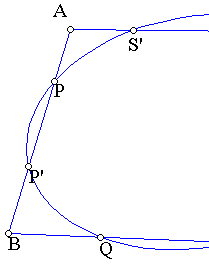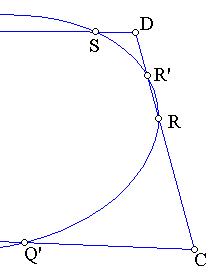##1. Carnot's theorem

Let the sides {AB,BC,CD} of triangle ABC intersect the conic c at points respectively P,P', Q,Q', R,R'. Then the following relation is valid.
AP*AP'*BQ*BQ'*CR*CR' = AR'*AR*CQ'*CQ*BP'*BP.
The relation is also sufficient i.e. if it is satisfied then there is a conic passing through the six points {P,P',Q,Q',R,R'}.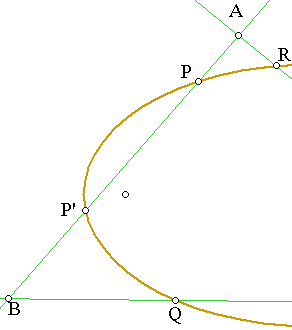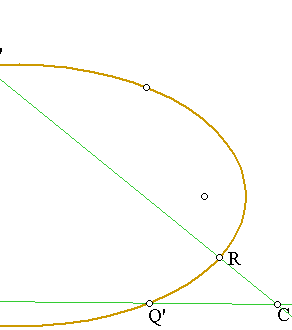The relation follows by noticing that the ratios of the kind:
(AP*AP')/(AR*AR')   =    I(AC)/I(AB)                                (*).
Where I(AB) is a quadratic form depending only on the direction of line AB: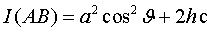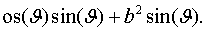Here it is assumed that the conic is represented by an equation of the form: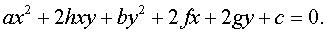Also line AB is assumed to be parameterized by the equation (variable r):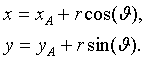These relations were established in the discussion of the generalization of  the power of a point with respect to a conic (See PowerGeneral.html ). They can be used here by applying (*)  successively three times for the lines through {A,B,C} respectively:
(AP*AP')/(AR*AR')    =    I(AC)/I(AB),
(BQ*BQ')/(BP*BP')     =   I(AB)/I(BC),
(CR*CR')/(CQ*CQ')    =   I(BC)/I(AC).
The result follows by multiplying the three equalities and observing that the resulting right side equals to 1.
To prove the converse consider the conic passing through five of the six points and use the proven part and the valid relation to show that the sixth point is also on that conic.

##2. Some consequences of Carnot's theorem

I follow here the remarks by Poncelet (treatise vol.1 p. 18). Carnot's theorem has many consequences. First consider the limit case in which the triangle becomes circumscribed about the conic. Then the picture becomes: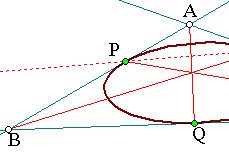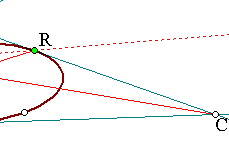and the relation becomes (simplifying squares and taking orientation into account).
AP*BQ*CR = - AR*CQ*BP.
This is Ceva's ( Ceva.html ) theorem and implies that  the lines joining the contact points with the opposite vertices are concurrent .
Assume now that the chord of contacts PR is parallel to BC. Then AP/BP = AR/CR implies that Q is the middle of BC.
Hence, by the parallelity AQ passes through the middle of PR. By drawing a tangent  B'C' parallel to BC we see that its middle Q' is also on line AQ.
The result is the well known property of conjugate diameters of conics.
Chords parallel to a given direction (BC) have their middles on another chord (QQ') passing through the contact points of BC and its parallel B'C'. Besides the tangents at the contact points (P,R) of the parallel chords intersect at points (A) lying on "conjugate" diameter QQ' of the conic.

##3. Extension of Carnot's theorem

The reasoning adopted in 1. can be extended to arbitrary polygons (with n sides) intersecting a conic. For example, a quadrilateral ABCD intersecting the conic at points P,P', Q,Q', ... etc. would produce the relation.
(AP*AP')*(BQ*BQ')*(CR*CR')*(DS*DS') = (AS*AS')*(DR*DR')*(CQ*CQ')*(BP*BP').